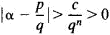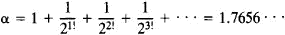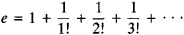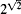# transcendental number

(redirected from Transcendental numbers)
Also found in: Dictionary, Thesaurus.

## transcendental number:

see numbernumber,
entity describing the magnitude or position of a mathematical object or extensions of these concepts. The Natural Numbers

Cardinal numbers describe the size of a collection of objects; two such collections have the same (cardinal) number of objects if their
.

## Transcendental Number

a real or imaginary number that is not algebraic. In other words, a transcendental number is a number that does not satisfy any algebraic equation with integer coefficients.

The existence of transcendental numbers was first established by J. Liouville in 1844. Liouville’s starting point was his theorem stating that the order of approximation of a given algebraic irrational number by a rational fraction with a given denominator cannot be made arbitrarily high. More precisely, if an algebraic number α satisfies an irreducible nth-degree algebraic equation with integer coefficients, then there exists a number c such thatfor any rational number p/q; here, c depends only on α. Therefore, the given irrational number α is transcendental if an infinite set of rational approximations can be found that do not satisfy the above inequality for any c and n (which are the same for all the approximations). An example of such a number isAnother proof of the existence of transcendental numbers was given by G. Cantor in 1874. He showed that the set of algebraic numbers is countable—that is, the algebraic numbers may be put into a one-to-one correspondence with the set of positive integers (seeSET THEORY). By contrast, the set of all real numbers is not countable. Consequently, the set of transcendental numbers is not countable; moreover, the transcendental numbers make up the bulk of the set of all numbers.

A fundamental problem in the theory of transcendental numbers is to determine whether the values of analytic functions with particular arithmetic and analytic properties are transcendental when the argument has algebraic values. Problems of this kind rank with the most difficult in modern mathematics. In 1873, C. Hermite proved that the base of the natural logarithmsis transcendental. The German mathematician F. Lindemann obtained in 1882 a more general result: if α is an algebraic number, then eα is a transcendental number. In 1930, Lindemann’s result was extended considerably by the German mathematician K. Siegel, who demonstrated, for example, the transcendence of the values of a broad class of cylindrical functions for algebraic values of the arguments.

At a mathematical congress in Paris in 1900, D. Hilbert listed 23 unsolved mathematical problems. One of them was whether the number αβ is transcendental if α and β are algebraic numbers and β is an irrational number. In particular, the question arises whether the numbersand eπ are transcendental. It may be noted that the problem of the transcendence of numbers of the type αβ was first stated in a special form by L. Euler in 1744. A complete solution of the problem was not obtained until 1934, when A. O. Gel’fond showed that such numbers are transcendental. From Gel’fond’s discovery it follows, in particular, that all common logarithms of natural numbers are transcendental.

Methods of the theory of transcendental numbers have been applied to a number of problems associated with the integer solution of equations.

### REFERENCE

Gel’fond, A. O. Transtsendentnye i algebraicheskie chisla. Moscow, 1952.

## transcendental number

[¦tran‚sen¦dent·əl ′nəm·bər]
(mathematics)
An irrational number that is the root of no polynomial with rational-number coefficients.
Site: Follow: Share:
Open / Close